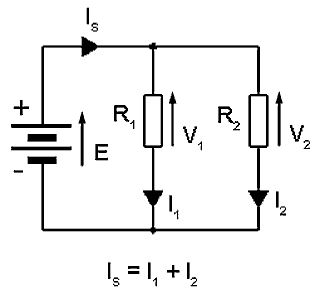# Current & Voltage

• After studying this section, you should be able to:
• Describe the distribution of electrical potentials (voltages) and currents in electrical circuits.
• •Series resistive circuits.
• •Parallel resistive circuits.
• Calculate the distribution of voltages in a resistive potential divider.

## Current & Voltage in Resistor Networks

### Finding the Unknown

In addition to working out the resistance, Ohms law can be used to work out voltages and currents in resistor networks. Before trying this it would be a good idea to look at some basic facts about resistor networks.### Fig.4.0.1 A Simple Series Circuit### Fig.4.0.2 A Simple Parallel Circuit

In the simple SERIES CIRCUIT shown in Fig. 4.0.1 the same current flows through all components. Each component however, will have a different VOLTAGE (p.d.) across it. The sum of these individual voltages (VR1+VR2+VR3 etc) in a series circuit is equal to the supply voltage (EMF).

In the simple PARALLEL CIRCUIT shown in Fig 4.0.2 however, the same voltage is present across all components but a different CURRENT can flow through each component. The sum of these individual component currents in a parallel circuit is equal to the supply current. (IS = IR1+ IR2+ IR3 etc.)

### The Potential Divider Rule### Fig. 4.0.3 A Potential Divider

If two or more resistors are connected in series across a potential (e.g. A supply voltage), the voltage across each resistor will be proportional to the resistance of that resistor. VR1 ∝ R1 and VR2 ∝ R2 etc.

To calculate the voltage across any resistor in the potential divider, multiply the supply voltage (E) by the proportion of that resistor to the total resistance of all the resistors.

For example if R2 is double the value of R1 there will be twice the voltage across R2 than across R1. It follows therefore, that the voltage across R1 will be one third of the supply voltage (E) and the voltage across R2 will be two thirds of the supply voltage (E). So, if the supply voltage and the resistor values are known, then the voltage across each resistor can be worked out by PROPORTION, and once the voltage across each resistor is known the voltage at any point in the circuit can be calculated.

Using these few facts it is possible to work out an amazing amount of information about the currents and voltages in a circuit, once the values of the circuit resistances are known. Try it out for yourself with our Quiz in the Resistors & Circuits Module 4.6 Network Calculations page.